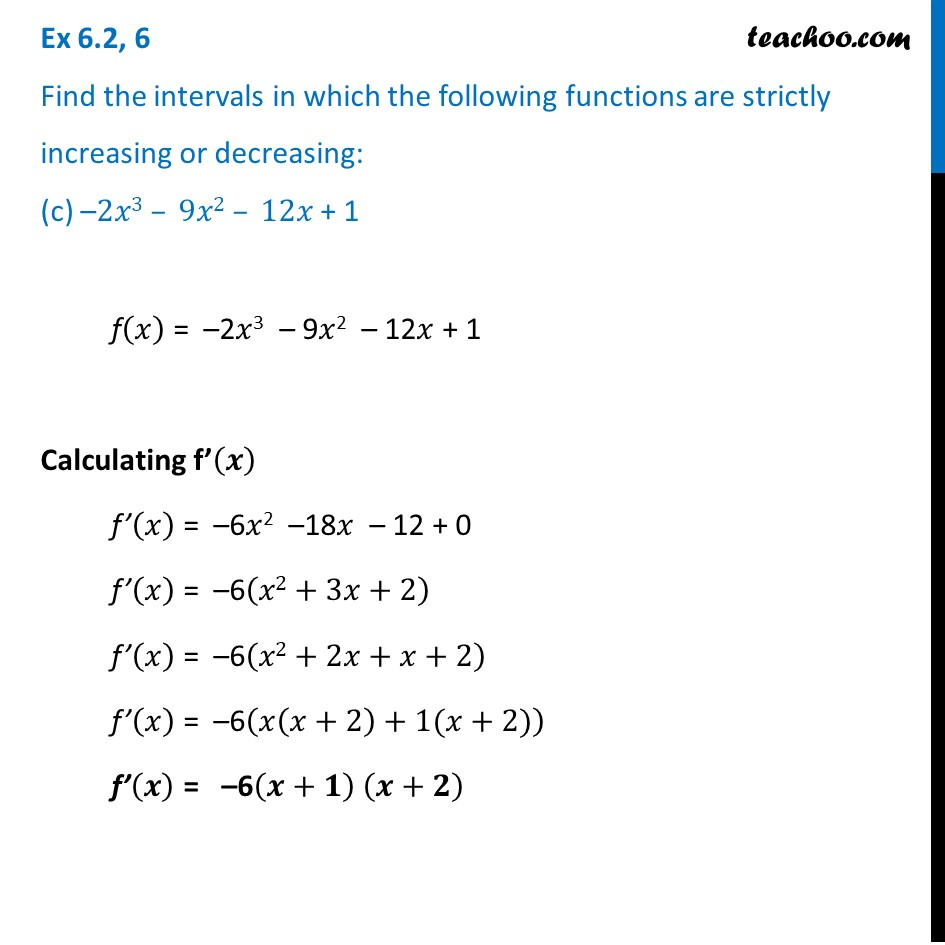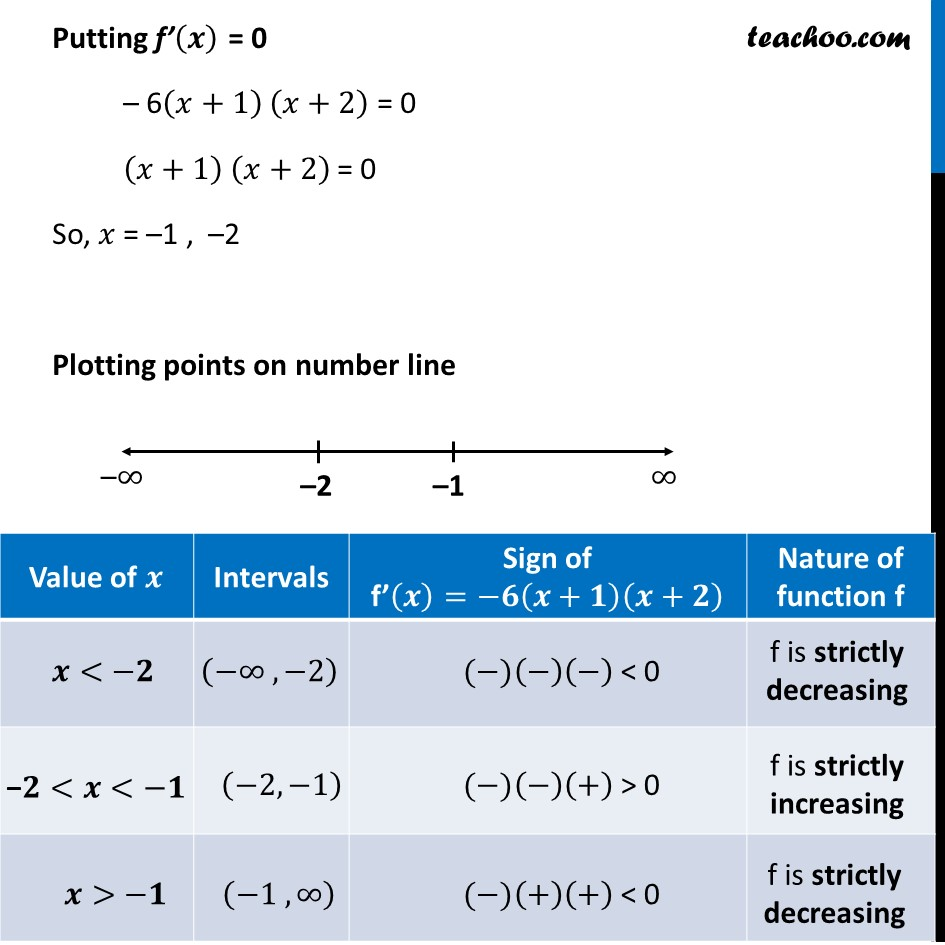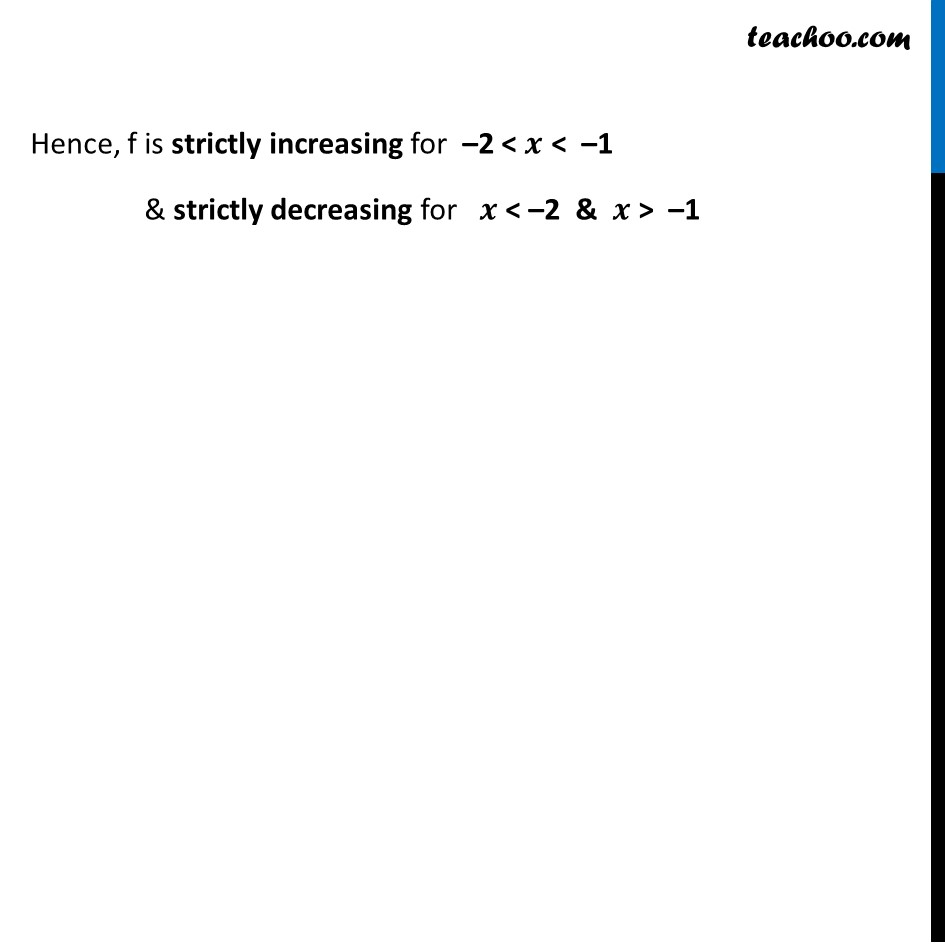Ex 6.2

Chapter 6 Class 12 Application of Derivatives
Serial order wiseLearn in your speed, with individual attention - Teachoo Maths 1-on-1 Class

### Transcript

Ex 6.2, 6 Find the intervals in which the following functions are strictly increasing or decreasing: (c) –2𝑥3 – 9𝑥2 – 12𝑥 + 1 f(𝑥) = –2𝑥3 – 9𝑥2 – 12𝑥 + 1 Calculating f’(𝒙) f’(𝑥) = –6𝑥2 –18𝑥 – 12 + 0 f’(𝑥) = –6(𝑥2+3𝑥+2) f’(𝑥) = –6(𝑥2+2𝑥+𝑥+2) f’(𝑥) = –6(𝑥(𝑥+2)+1(𝑥+2)) f’(𝒙) = –6(𝒙+𝟏) (𝒙+𝟐) Putting f’(𝒙) = 0 – 6(𝑥+1) (𝑥+2) = 0 (𝑥+1) (𝑥+2) = 0 So, 𝑥 = –1 , –2 Plotting points on number line Hence, f is strictly increasing for –2 < 𝒙 < –1 & strictly decreasing for 𝒙 < –2 & 𝒙 > –1 Hence, f is strictly increasing for –2 < 𝒙 < –1 & strictly decreasing for 𝒙 < –2 & 𝒙 > –1